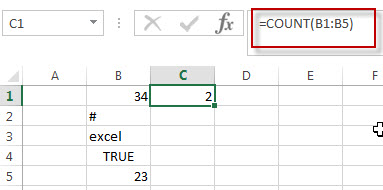# Excel COUNT Function

This post will guide you how to use Excel COUNT function with syntax and examples in Microsoft excel.

## Description

The Excel COUNT function counts the number of cells that contain numbers, and counts numbers within the list of arguments. It returns a numeric value that indicate the number of cells that contain numbers in a range.

The COUNT function is a build-in function in Microsoft Excel and it is categorized as a Statistical Function.

The COUNT function is available in Excel 2016, excel 2013, excel 2010, excel 2007, excel 2003, excel XP, excel 2000, excel 2011 for Mac.

## Syntax

The syntax of the COUNT function is as below:

`= COUNT(value1, [value2],…)`

Where the COUNT function arguments are:

• value1 -This is a required argument.  The first item, cell reference, or range within which you want to count numbers.
• Value2 – This is an optional argument. You can enter up to 255 value arguments.

Note:

• Arguments that are numbers, dates, or a text representation of numbers (for example, a number enclosed in quotation marks, such as “1”) are counted.
• If the argument is an array or reference, only numbers in that array are counted. Empty cells, logical values, text, or error values in the array or reference are not counted.
• You can use the COUNTA function to count logical values, text, error values.
• You can use the COUNTIF function to count only numbers that meet certain criteria.
• You can provide up to 255 number arguments in excel 2007 or later. If you are using excel 2003, you can only provide up to 30 number arguments.

## Excel COUNT Function Examples

The below examples will show you how to use Excel COUNT Function to count the number of cells that contain numbers in a range.

#1 To count the number of cells in the range B1:B4, just using the following excel formula:

=COUNT(B1:B4)